Definition: irrational number from Philip's Encyclopedia

In mathematics, any number that cannot be expressed as the ratio of two integers. An example is √2: like other irrational numbers, its expression as a decimal is infinite and non-repeating. Irrational numbers, together with the rational numbers, make up the set of real numbers.

Summary Article: III.41 Irrational and Transcendental Numbers
From The Princeton Companion to Mathematics

An irrational number is one that cannot be written as a/b with both a and b integers. A great many naturally occurring numbers, such as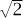, e, and π, are irrational. The following proof thatis irrational is one of the best-known arguments in all of mathematics. Suppose that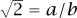since common factors can be canceled, we may assume that a and b have no common factor; we have a2 = 2b2, which means that a must be even; write a = 2c; but then 4c2 = 2b2, which implies that 2c2 = b2, and hence b must be even too; this, however, is contrary to our assumption that a and b were coprime.

There are several famous conjectures in mathematics that ask whether certain specific numbers are rational or not. For example, π + e and πe are not known to be irrational, and neither is Euler’s constant: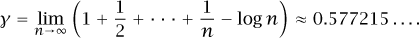It is known that ζ(3) = 1 + 2−3 + 3−3 + . . . is irrational. Almost certainly, ζ(5), ζ(7), ζ(9), . . . are all irrational as well. However, although it has been shown that infinitely many of these numbers are irrational, no specific one is known to be.

A classic proof is that of the irrationality of e. If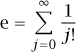were equal to p/q, then we would have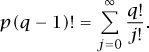The left-hand side and the terms of the sum with j ≤ q are all integers. Therefore the quantity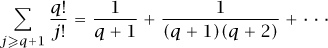is also an integer. But it is not hard to show that this quantity lies strictly between 0 and 1, a contradiction.

The principle used here, that a nonzero integer must have absolute value at least one, is surprisingly powerful in the theory of irrational and transcendental numbers.

Some numbers are more irrational than others. In a sense, the most irrational number is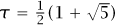, the golden ratio, because the best rational approximations to it, which are ratios of consecutive Fibonacci numbers, approach it rather slowly. There is also a very elegant proof that τ is irrational. This is based on the observation that the τ × 1 rectangle R may be divided into a square of side 1 and a 1 × 1 rectangle. If τ were rational, then we would be able to create a rectangle with integer sides that was similar to R. From this we could remove a square, and we would be left with a smaller rectangle with integer sides that would still be similar to R. We could continue this process ad infinitum, which is clearly impossible.

A transcendental number is one which is not algebraic, that is to say, is not the root of a polynomial equation with integer coefficients. Thus,is not transcendental, since it solves x2 − 2 = 0, and neither is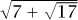.

Are there, in fact, any transcendental numbers? This question was answered by liouville [VI.39] in 1844, who showed that various numbers were transcendental, of which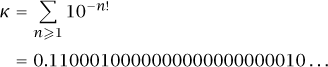is a well-known example. This is not algebraic, because it can be approximated by rationals more accurately than any algebraic number can. For example, the rational approximation 110 001/1 000 000 is very close indeed to κ, but its denominator is not particularly large.

Liouville showed that if α is a root of a polynomial of degree n, then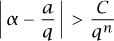for all integers a and q and for some constant C depending on α. In words, α cannot be too well approximated by rationals. Roth later proved that the exponent n here can actually be replaced by 2 + ε for any ε > 0. (For more on these topics, see liouville’s theorem and roth’s theorem [V.22].)

A completely different approach to the existence of transcendental numbers was discovered by cantor [VI.54] thirty years later. He proved that the set of algebraic numbers is countable [III.11], which means, roughly speaking, that they may be listed in order. More precisely, there is a surjective map from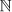, the set of natural numbers, to the set of algebraic numbers. By contrast, the real numbers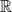are not countable. Cantor’s famous proof of this uses a diagonalization argument to show that any listing of all the real numbers must be incomplete. There must, therefore, be real numbers that are not algebraic.

It is generally rather difficult to prove that a specific number is transcendental. For instance, it is by no means the case that all transcendental numbers are very well approximated by rationals; this merely provides a useful sufficient condition. There are other ways to establish that numbers are transcendental. Both e and π are known to be transcendental, and it is known that |e − a/b| > C(ε)/b2 + ε for all ε > 0, so e is not all that well approximated by rationals. Since ζ(2m) is always a rational multiple of π2m, it follows that the numbers ζ(2), ζ(4), . . . are all transcendental.

The modern theory of transcendental numbers contains a wealth of beautiful results. An early one is the Gel’fond–Schneider theorem, which says that αβ is transcendental if α ≠ 0, 1 is algebraic, and if β is algebraic but not rational. In particular,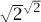is transcendental. There is also the six-exponentials theorem, which states that if x1, x2 are two linearly independent complex numbers, and if y1, y2, y3 are three linearly independent complex numbers, then at least one of the six numbers ex1y1, ex1y2, ex1y3, ex2y1, ex2y2, ex2y3 is transcendental. Related to this is the (as yet unsolved) four-exponentials conjecture: if x1 and x2 are two linearly independent complex numbers, and if y1 and y2 are linearly independent, then at least one of the four exponentials ex1y1, ex1y2, ex2y1, ex2y2 is transcendental.Copyright © 2010 by Princeton University Press

### Related Articles

##### Full text Article III.41 Irrational and Transcendental Numbers
The Princeton Companion to Mathematics

An irrational number is one that cannot be written as a/b with both a and b integers. A great many naturally occurring numbers, such as ...

##### Full text Article Algebraic Numbers
Bloomsbury Guide to Human Thought

In the development of numbers , algebraic numbers come in generality between the rational numbers and the real numbers . A rational number...

##### Full text Article Rational Numbers
Bloomsbury Guide to Human Thought

A rational number, in mathematics, is one which can be represented by a fraction, the ratio of two integers (the one which does the...

See more from Credo